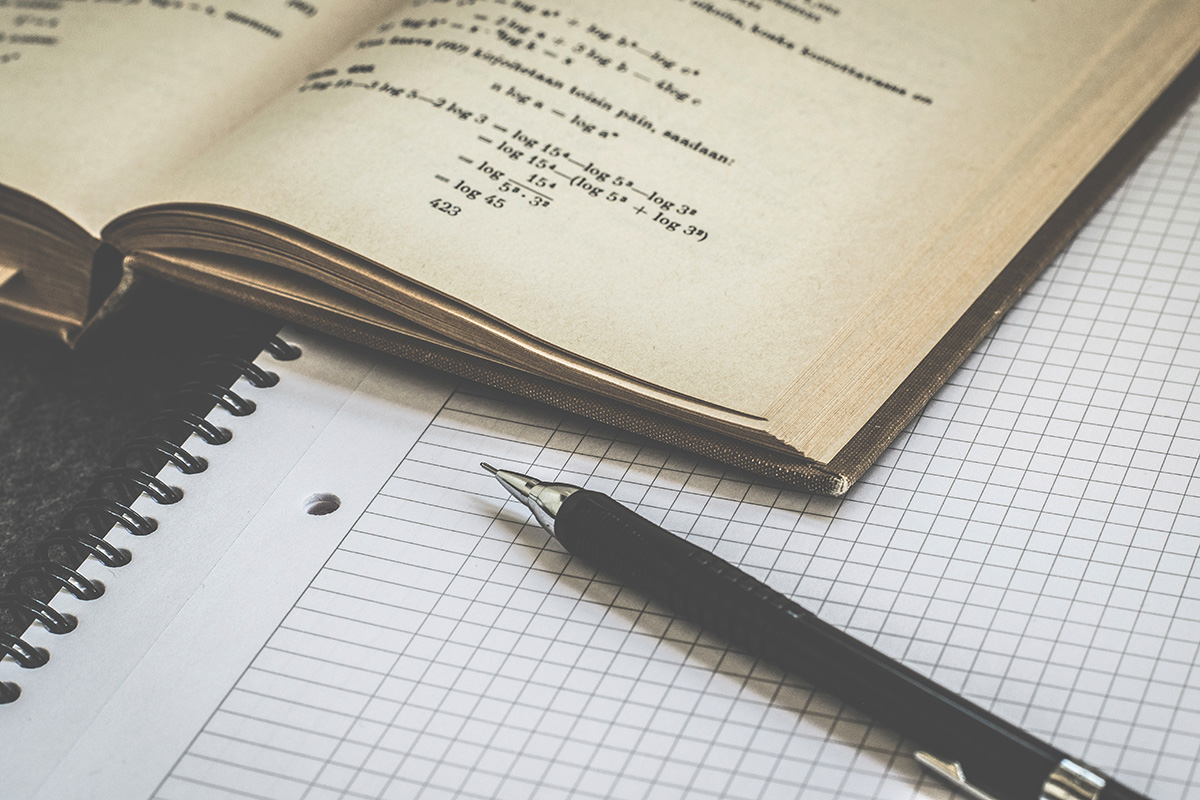This is a quick cheat sheet of different ways to calculate area and volume based on shape.

## Areas Calculations

### Area of a Circle

$A = \pi r^2$

So for example, if we had a Circle with a diameter of 8 inches, and we want to know the area of the circle, we would first need the radius. The radius is half the diameter, in this case it would be 4 inches.

Now that we know the radius, we can plug it into the equation:

$A = \pi 4^2$

4 squared is 16 (4×4), so that means we have:

$A = \pi \times 16$ We could say $16\pi$

We could also calculate this, using whatever cut off of PI that we choose… i.e. 3.14 or 3.14159265359 and multiply that by 16.

### Circumference of a Circle

To find the length of the outer edge of the circle, we use a different equation:

$C = 2\pi r$

In this case we need the radius, and multiple it by 2 and then multiple that by PI.

But what if we need to find the radius and all we know is the circumference?

Transforming the equation above, we can get:

$r = {C \over 2\pi}$

Transforming equations isn’t that hard, if you remember we have to do the same thing to each side of the = sign. In this case we divided out 2pi from the original equation, leaving r by itself, and also dividing C by 2pi.

Now, if we know the circumference, we can find the radius.

### Area of a Rectangle

This is easy, you take the length and multiple it by the width. BUT REMEMBER TO CHANGE YOUR UNITS TO UNITS SQUARED! So if the length is 8ft and the width is 2ft, then the area is 16ft squared.

### Area of a Triangle

The formula for this is to get:

${1\over 2}bh$

If the base is 8cm, then half the base is 4, and we multiple that by the height. If the height were 3, then the area would be 12cm squared.

## Area of a parallelogram

To find the area of a parallelogram, you need the base times the height. You do not use the height of the leaning sides at all, you only need the base length and the height.

## Area of a trapezoid

A trapezoid can be imagined as a mountain in the shape of a triangle with its top cut off. A trapezoid contains two bases unlike the usual one in most shapes. To find the area of a trapezoid, you add the bases together, and multiply it by the height.

## Surface area of a prism

The surface area of a prism is like a UV Unwrap in Blender. Meaning each side’s area is considered as a total surface area. This is different from volume, which is the amount of space inside the 3D prism.

$A = 2B + Ph$

The equation for this is 2 X the area of the base plus the permitter of the base X the height of the prism. So if the prism has a square base of 4 x 4, then the area of the base is 16, and we must account for both ends, so we get 2 x 16 which equals 32. Next we take the permitter of the base (4 + 4 + 4 + 4 = 16). So the permitter is also 16. If the height is 8, then when calculating the second part (permitter times height), or 8 X 16 which is 128. We add the two together now, 32 + 128, to get 160.

If the prism is a triangular prism, then the Base would be the area of a triangle (instead of a rectangle).

## Volume of a Prism

To calculate the space within a 3D shape, we use a different equation:

$V = Bh$

B is the area of the base. First, find the area of whatever base shape it is. Then multiply that by the height of the prism. The result is the volume of the object.

Categories:

Tags: